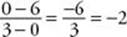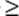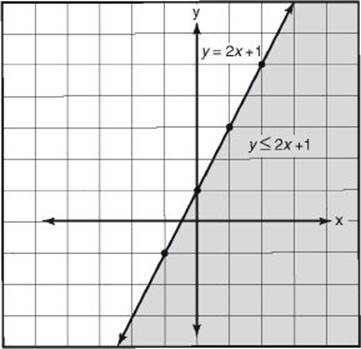﻿ ﻿EQUATIONS OF LINES - Coordinate Geometry - SOLID AND COORDINATE GEOMETRY - SAT SUBJECT TEST MATH LEVEL 1

## SOLID AND COORDINATE GEOMETRY## CHAPTER 13Coordinate Geometry### EQUATIONS OF LINES

Every line that is drawn in a coordinate plane has an equation. A point (x, y) is on a line if and only if the values of x and y satisfy that equation. All the points on a horizontal line have the same y-coordinate. For example, in the figure below, horizontal linepasses through (–3, 4), (0, 4), (2, 4), (5, 4), and (10, 4). The equation of lineis y = 4. Similarly, every point on a vertical line has the same x-coordinate. Each point on line m has an x-coordinate of 3. The equation of m is x = 3.Every other line in the coordinate plane has an equation that can be written in the form y = mx + b, where m is the slope of the line and b is the y-intercept—the y-coordinate of the point where the line crosses the y-axis. These facts are summarized in KEY FACT L8.

Key Fact L8

For any real number a, x = a is the equation of the vertical line that crosses the x-axis at (a, 0).

For any real number b, y = b is the equation of the horizontal line that crosses the y-axis at (0, b).

For any real numbers b and m, y = mx + b is the equation of the line that crosses the y-axis at (0, b) and whose slope is m.

On the Math 1 test, you could be given an equation and asked which of five graphs is the graph of that equation. Conversely, you could be given a graph and asked which of five equations is the equation of that graph.

EXAMPLE 7: Which of the following is the equation of the line in the figure below?(A) y = –2x + 6

(B) y = –x + 6

(C) y = 2x + 6

(D) y = –2x – 6

(E) y = 2x – 6

On the Math 1 test, there are two ways to answer the question:

Solution 1. Since the line is neither horizontal nor vertical, its equation has the form y = mx + b. Since the line crosses the y-axis at 6, b = 6. Since the line passes through (0, 6) and (3, 0), its slope, m, is. The equation is y = –2x + 6, choice A.

Solution 2. The second way to answer this question takes advantage of the fact that the Math 1 test is a multiple-choice test. Since the line passes through (0, 6), y = 6 when x = 0. Plug in 0 for x in the five choices, and eliminate choices D and E, the two choices for which y6. Then note that the line also passes through (3, 0), so when x = 3, y = 0. Replace x by 3 in the remaining choices (A, B, and C) and see which one equals 0. Only choice A works.

EXAMPLE 8: What is the equation of the line that passes through (1, –3) and (5, 5)?

First find the slope of the line:So the equation is y = 2x + b.

To find b, replace x and y by the x- and y-coordinates of either of the two given points, say (5, 5):The equation is y = 2x – 5.

Any equation in which the only variables are x and y, and neither x nor y is written with an exponent, is the equation of a line.

EXAMPLE 9: 3y – 4x + 6 = 0 is the equation of a line. To determine the slope and y-intercept of this line, first solve for y :The equation is now written in the form y = mx + b. We can see that the slope isand the y-intercept is –2.

In order to write the equation of a line, you need to know two things: the slope of the line and a point on the line. If the point you know happens to be the y-intercept, then, of course, you can use the equation y = mx + b. If the point is not the y-intercept, you have two choices. The first is to use the equation y = mx + b and proceed as in Example 8. The second is to use the equation given in KEY FACT L9.

Key Fact L9

The equation of the line that passes through (x1, y1) and has slope m is yy1= m(xx1).

EXAMPLE 10: Line n passes through (1, 1) and is parallel to line, whose equation is y = 2x – 3. What is the equation of n?

Note that the slope ofis 2, and since parallel lines have equal slopes, the slope of n is also 2. So the equation of n is:

y – 1 = 2(x – 1)y – 1 = 2x – 2y = 2x – 1

Occasionally on the Math 1 test, there is a question concerning a linear inequality, such as y > 2x + 1. The graph of this inequality consists of all the points that are above the line y = 2x + 1. Note that the point (2, 5) is on the line, whereas (2, 6), (2, 7), and (2, 8) are all above the line. We indicate the set of all points satisfying the inequality by shading or striping the region above the line. To indicate that the points on the line itself do not satisfy the inequality, we draw a dotted line. To indicate that the points on a line are included in a graph, we draw a solid line. For example, (2, 5) is not on the graph of y > 2x + 1, but it is on the graph of y2x + 1. Similarly, the graph of y < 2x + 1 and y2x + 1 are shaded or striped regions below the line y = 2x + 1. These inequalities are shown in the following graphs.﻿